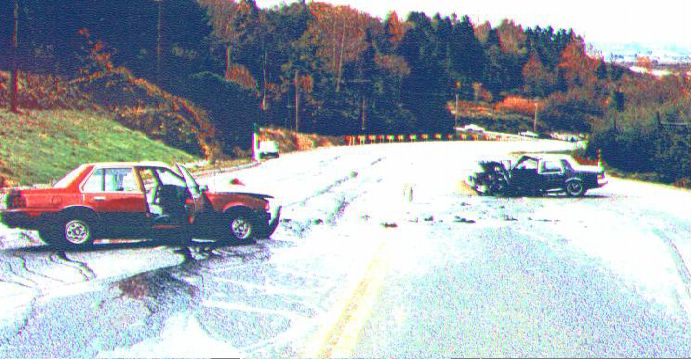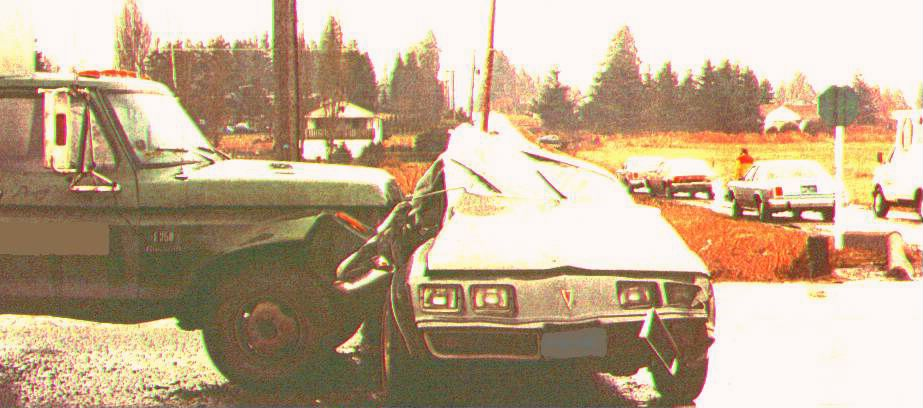An Example

There are huge number of different collisions to consider.To simplify things, I'll stick with a linear collision.

A Ford pickup truck is travelling east at an unknown velocity. A Pontiac Firebird reaches the intersection. Believing that he has enough time, he crosses the road, heading south.

As the car is about to leave the intersection, a child, who was behind some parked cars and which was unseen by the driver of car, crosses in front of the car. This forces the car to stop while still in the intersection.

For a reason unknown, the truck never brakes. This may have been because the driver never applied the brakes, or because the brakes didn't work. Regardless, an officer at the scene is interested in discovering the velocity of the truck before the collision.The Ford pickup truck slams into the stationary Pontiac Firebird. The two vehicles are in an inelastic collision. They leave skid marks that are 22.5 m long. How fast was the truck traveling before impact?

Each vehicle had a driver only. We'll assume that each driver was about 75 kg. The mass of the truck (with load) is 2722 kg, and the mass of the car is 1556 kg. Adding the mass of the drivers gives:

Truck : 2797 kg Car : 1631 kg

The coefficient of friction of the car's tires was found to be =0.47

Retarding force of the car, Fr = 0.47 Fn = 0.47( m g)

= 0.47 (1631)(9.81)

= 7520 N

Conservation of momentum

m1 v1 + m2v2 = (m1 + m2)v

2797 v1 + 0 = (2797 + 1631)v

v1 = 1.58 v

a = -Fr / m ( there's a minus sign because F opposes motion)

= - 7520 /(2797 + 1631) = - 1.70 m/s^2

V^2 = v^2 + 2 a d, V final = 0

v^2 = - 2 ( - 1.70)(22.5) = 76.5

v = 8.75 m/s

initial v = 1.58 ( 8.75) = 13.8 m/s = 49.7 km/hr

The minimum velocity of the truck was about 50 km/hr, which was the posted speed limit.

If someone is interested in having an idea of the chance of survival of this collision, one could use the Severity Index.

V^2 = v^2 + 2 a d, a = g,

As I mentioned earlier, a automobile travelling at 50 km/hr undergoes an average acceleration of 15 g. The majority of the crumpling of the vehicles occurs at the very begining of the collision. To facilitate things, we'll have to make an approximation. That is that the amount of crumpling from both vehicles would be approximate to that of one vehicle hitting a wall. To find the distance crumpled

final V = 0, initial v = 13.8 m/s

d = -v^2 / 2 a = - (13.8)^2 / 2 (15 (9.81)) = - 0.647 m

But this is for the wall, and the direction would have to be into the car. All we want is the absolute of this 0.647 m

Crumple time

V = v + a t , final V = 0 ; t = (V - v )/ a = (8.75 - 13.8) / 15 (9.81)

= 0.0343 s

For Truck

S.I. = (alpha)^5/2 t = (15)^5/2 (0.0343) = 29.9 A driver wearing a seatbelt should survive.

The stopping distance of a human is about 1/15th of that of a car.

If we work out the Severity Index in terms of crumple distance and velocity

= a / g ; V^2 = v^2 + 2 a d ; final V = 0

a = - v^2 / 2 do (we're just interested in magnitudes) = v^2 / 2 do

The crumple distance of a person is given as 1/15th of car

do = d / 15

SI = (alpha)^5/2 t = (a^5/2 / g^5/2) t = (a^5/2 / g^5/2) v / a = (a^3/2 / g^5/2) v

= (v^2 / (2 d/ 15))^3/2 v = v^4 / ( (2 d / 15)^3/2 (g)^5/2)

= 4748 > 1000; A driver not wearing a seatbelt would not likely survive.

For Car

a = (V - v ) / 2 t = (8.75 ) / 2 (0.0343) = 128 m/s2 = 13.0 g

S.I. = (alpha)^5/2 t = (13)^5/2 (0.0343) = 20.9 A driver wearing a seatbelt should survive.

S.I. = v^4 / ( (2 d / 15)^3/2 (g)^5/2)= 768 which is > 1000

A driver not wearing a seatbelt would likely survive..